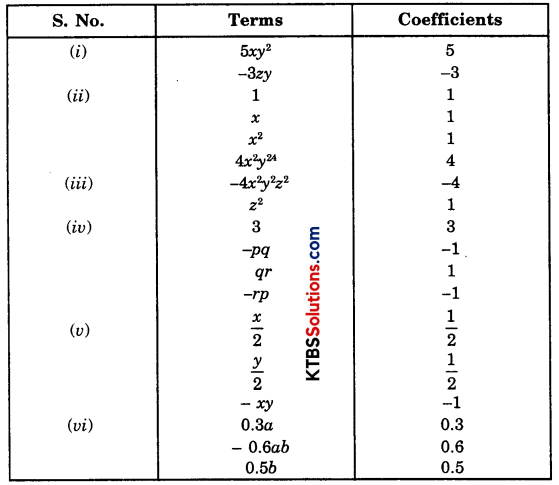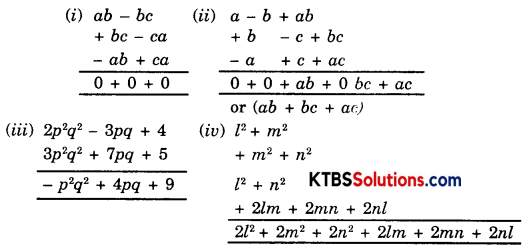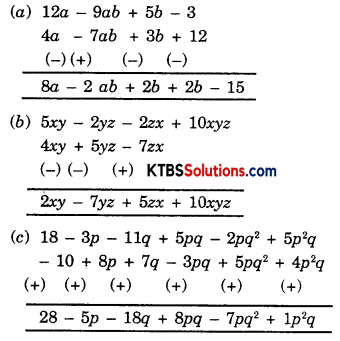# KSEEB Solutions for Class 8 Maths Chapter 9 Algebraic Expressions and Identities Ex 9.1

You can Download KSEEB Solutions for Class 8 Maths Chapter 9 Algebraic Expressions and Identities Ex 9.1 Questions and Answers helps you to revise the complete syllabus.

## KSEEB Solutions for Class 8 Maths Chapter 9 Algebraic Expressions and Identities Ex 9.1

Question 1.
Identify the terms, their coefficients for each of their following expressions.
(i) 5xy2 – 3zy
(ii) 1 + x + x2
(iii) 4x2y2 – 4x2y2z2 – z2
(iv) 3 – pq + qr – rp
(v) $$\frac{x}{2}+\frac{y}{2}$$ – xy
(vi) 0.3a – 0.6ab + 0.5b
Solution:Question 2.
Classify the following polynomials as monomials, binomials, trinomials. Which polynomials do not fit in any of these three categories?
x + y, 100, x + x2 + x3 + x4, 7 + y5x, 2y – 3y2, 2y – 3y2 + 4y3, 5x – 4y + 3xy, 4z – 15z2, ab + bc + cd + da, pqr, p2q + pq2, 2p + 2q
Solution:
Monomials = 100, pqr
Binomials = x + y, 2y – 3y2, 4z – 15z2, p2q + pq2, 2p + 2q
Trinomials = 7 + y 5x, 2y – 3y2, 4y3, 5x – 4y + 3xy
Polynomials = x + x2 + x3 + x4, ab + bc + cd + daQuestion 3.